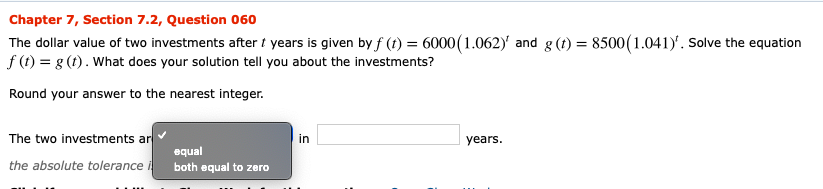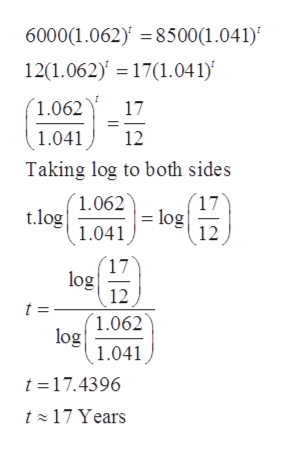# Chapter 7, Section 7.2, Question 060The dollar value of two investments after t years is given by f (t) = 6000(1.062)' and g (t) = 8500 (1.041). Solve the equationf (t) g (t). What does your solution tell you about the investments?Round your answer to the nearest integerThe two investments arinyears.equalthe absolute tolerance iboth equal to zero

Question
12 viewshelp_outlineImage TranscriptioncloseChapter 7, Section 7.2, Question 060 The dollar value of two investments after t years is given by f (t) = 6000(1.062)' and g (t) = 8500 (1.041). Solve the equation f (t) g (t). What does your solution tell you about the investments? Round your answer to the nearest integer The two investments ar in years. equal the absolute tolerance i both equal to zero fullscreen
check_circle

Step 1

Given:

Step 2

According to the question f(t)...help_outlineImage Transcriptionclose6000(1.062) =8500(1.041) 12(1.062) 17(1.041 1.062 17 1.041 12 Taking log to both sides (1.062 t.log 1.041 17 - log 12 17 log 12 t = 1.062 log 1.041 t 17.4396 t 17 Years fullscreen

### Want to see the full answer?

See Solution

#### Want to see this answer and more?

Solutions are written by subject experts who are available 24/7. Questions are typically answered within 1 hour.*

See Solution
*Response times may vary by subject and question.
Tagged in

### Other